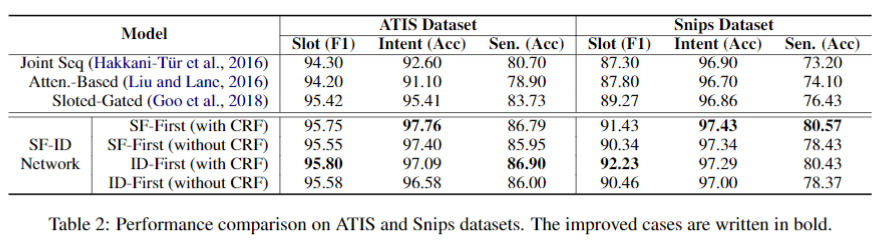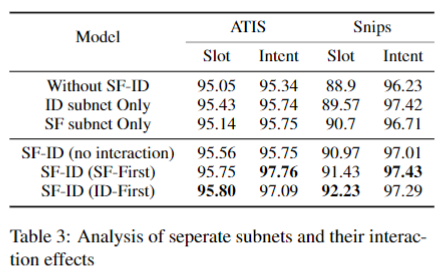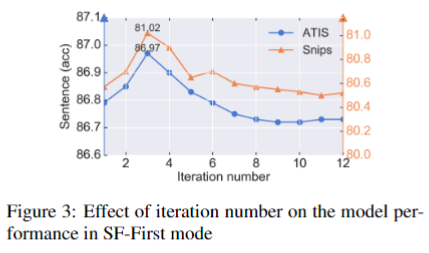# A Novel Bi-directional Interrelated Model for Joint Intent Detection and Slot Filling

source: ACL 2019 short paper
code: https://github.com/ZephyrChenzf/SF-ID-Network-For-NLU

## Models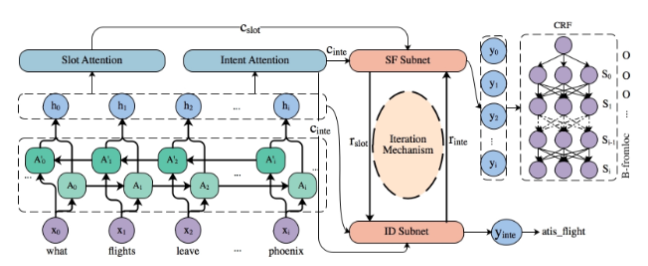$$c_{s l o t}^{i}=\sum_{j=1}^{T} \alpha_{i, j}^{S} h_{j}$$

### SF-ID Network

SF-ID Network 由两个模块组成：SF, ID。根据这两个模块执行顺序的不同，SF-ID Network可以分为两种：

• SF-First Mode
• ID-First Mode

### SF-First Mode

SF subnet 首先通过意图信息$c_{inte}$和槽信息$c_{slot}^{i}$计算一个相关因子$f$：
$$f=\sum V \ast \tanh \left(c_{\text {slot}}^{i}+W \ast c_{\text {inte}}\right)$$

ID subnet 将slot信息融合到意图识别任务中，作者认为混合的多粒度信息有助于ID：
$$r=\sum_{i=1}^{T} \alpha_{i} \cdot r_{s l o t}^{i} \\ \alpha_{i}=\frac{\exp \left(e_{i, i}\right)}{\sum_{j=1}^{T} \exp \left(e_{i, j}\right)} \\ e_{i, j}=W \ast \tanh \left(V_{1} \ast r_{\text {slot}}^{i}+V_{2} \ast h_{j}+b\right)$$

Iteration Mechanism 反过来，$r_{\text {inte}}$也可以被输入到SF subnet中重新计算$f$：
$$f=\sum V \ast \tanh \left(c_{s l o t}^{i}+W \ast r_{i n t e}\right)$$

$$y_{i n t e}=\operatorname{softmax}\left(W_{i n t e}^{h y} \operatorname{concat}\left(h_{T}, r_{i n t e}\right)\right) \\ y_{s l o t}^{i}=\operatorname{softmax}\left(W_{s l o t}^{h y} \operatorname{concat}\left(h_{i}, r_{s l o t}^{i}\right)\right)$$

### ID-First Mode

ID subnet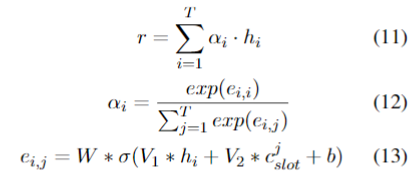## Experiment